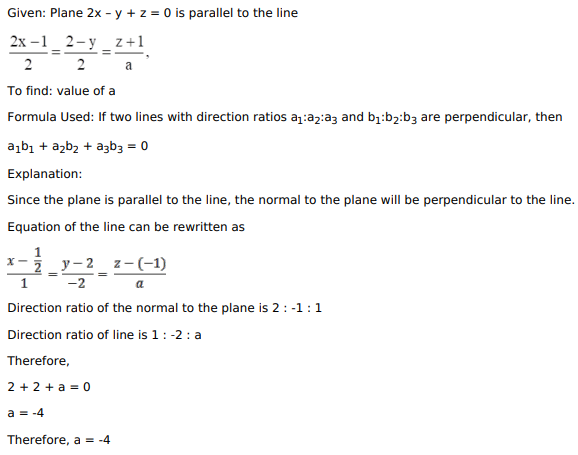# Mark against the correct answer in each of the following:

Question:

Mark against the correct answer in each of the following:

If the plane $2 x-y+z=0$ is parallel to the line $\frac{2 x-1}{2}=\frac{2-y}{2}=\frac{z+1}{a}$, then the value of $a$ is

A. $-4$

B. $-2$

C. 4

D. 2

Solution:Saniya
March 11, 2023, 7:29 p.m.
Hello my name is:-
Rubina
Sept. 16, 2022, 4:49 p.m.
Please sir science class X ke all lessons ke important notes bana dijiye
Kajal
Aug. 24, 2022, 5:31 p.m.
Sir ji aap mujhe science lessons ki revision krwa do you video sand kro youtube pr please 10th class
Joshua tolulope
May 31, 2022, 7:57 a.m.
You good sir.
Free Study Material